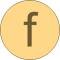# sort

Sort elements of vector in ascending or descending order# Information

This information is part of the Modelica Standard Library maintained by the Modelica Association.

#### Syntax

```           sorted_v = Vectors.sort(v);
(sorted_v, indices) = Vectors.sort(v, ascending=true);
```

#### Description

Function sort(..) sorts a Real vector v in ascending order and returns the result in sorted_v. If the optional argument "ascending" is false, the vector is sorted in descending order. In the optional second output argument the indices of the sorted vector with respect to the original vector are given, such that sorted_v = v[indices].

#### Example

```  (v2, i2) := Vectors.sort({-1, 8, 3, 6, 2});
-> v2 = {-1, 2, 3, 6, 8}
i2 = {1, 5, 3, 4, 2}
```

# Syntax

(sorted_v, indices) = sort(v, ascending)

# Inputs (2)

v Type: Real[:] Description: Real vector to be sorted Default Value: true Type: Boolean Description: = true if ascending order, otherwise descending order

# Outputs (2)

sorted_v Default Value: v Type: Real[size(v, 1)] Description: Sorted vector Default Value: 1:size(v, 1) Type: Integer[size(v, 1)] Description: sorted_v = v[indices]